## Type-2 Fuzzy Inference Systems

For any value in the universe of discourse, a traditional type-1 membership function has a single membership value. Therefore, while a type-1 membership function models the degree of membership in a given linguistic set, it does not model uncertainty in the degree of membership. To model such uncertainty, you can use interval type-2 membership functions. In such type-2 membership functions, the degree of membership can have a range of values.

For examples that use type-2 fuzzy inference systems, see Fuzzy PID Control with Type-2 FIS and Predict Chaotic Time Series Using Type-2 FIS.

### Interval Type-2 Membership Functions

An interval type-2 membership function is defined by an upper and lower membership function. The upper membership function (UMF) is equivalent to a traditional type-1 membership function. The lower membership function (LMF) is less than or equal to the upper membership function for all possible input values. The region between the UMF and LMF is the footprint of uncertainty (FOU). The following diagram shows the UMF (red) the LMF (blue), and the FOU (shaded) for a type-2 triangular membership function.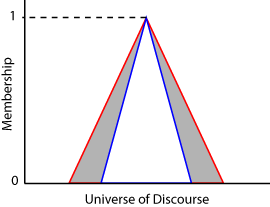For each input value in the universe of discourse, the degree of membership is the range of values between the LMF and UMF values.

### Type-2 Fuzzy Inference Systems

Using Fuzzy Logic Toolbox™ software, you can create both type-2 Mamdani and Sugeno fuzzy inference systems.

• In type-2 Mamdani systems, both the input and output membership functions are type-2 fuzzy sets.

• In type-2 Sugeno systems, only the input membership functions are type-2 fuzzy sets. The output membership functions are the same as for a type-1 Sugeno system — constant or a linear function of the input values.

To create type-2 Mamdani and Sugeno systems, use `mamfistype2` and `sugfistype2` objects, respectively. These objects have the same parameters as the type-1 `mamfis` and `sugfis` objects along with an additional `TypeReductionMethod` parameter.

You can also create a type-2 fuzzy inference system by converting an existing type-1 system, such as one created using the `genfis` function. To do so, use the `convertToType2` function.

Once you create a type-2 fuzzy inference system, you can:

### Fuzzy Inference Process for Type-2 Fuzzy Systems

#### Antecedent Processing

For type-2 fuzzy inference systems, input values are fuzzified by finding the corresponding degree of membership in both the UMFs and LMFs from the rule antecedent. Doing so generates two fuzzy values for each type-2 membership function. For example, the fuzzification in the following figure shows the membership value in the upper membership function (fU) and the lower membership function (fL).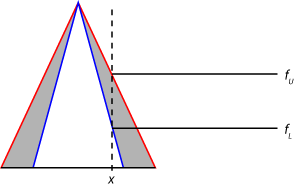Next, a range of rule firing strengths is found by applying the fuzzy operator to the fuzzified values of the type-2 membership functions, as shown in the following figure. The maximum value of this range (wU) is the result of applying the fuzzy operator to the fuzzy values from the UMFs. The minimum value (wL) is the result of applying the fuzzy operator to the fuzzy values from the LMFs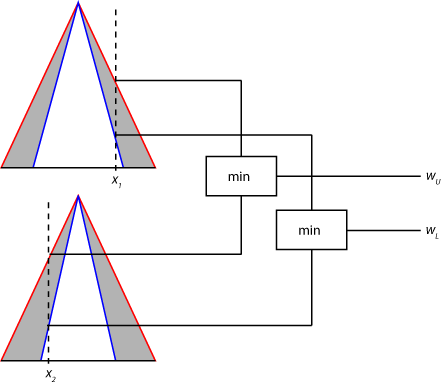Antecedent processing is the same for both Mamdani and Sugeno systems.

#### Consequent Processing

For a Mamdani system, the implication method clips (`min` implication) or scales (`prod` implication) the UMF and LMF of the output type-2 membership function using the rule firing range limits. This process produces an output fuzzy set for each rule. The following figure shows the output fuzzy set (dark gray region) produced by applying `min` implication to the UMF (red) and LMF (blue).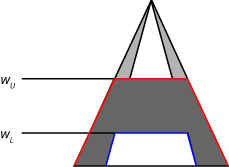For a type-2 Sugeno system, the output level zi for the ith rule is computed in the same manner as for a type-1 Sugeno system.

`${z}_{i}={c}_{0}^{i}+\sum _{j=1}^{M}{c}_{j}^{i}{x}_{j}$`

Here, j is the input index, xj is the value of the jth input variable, and the c terms are the upper membership function parameters

Unlike a type-1 Sugeno system, the rule firing strengths are not used to process the consequent of each rule. Instead, the output level and rule firing strengths are used during the aggregation process.

#### Aggregation

The goal of the aggregation stage is to derive a single type-2 fuzzy set from the rule output fuzzy sets.

For a type-2 Mamdani system, the software finds an aggregate type-2 fuzzy set by applying the aggregation method to the UMFs and LMFs of the output fuzzy sets of all the rules. The following figure shows the aggregation of two type-2 fuzzy sets (the outputs for a two-rule system) using `max` aggregation.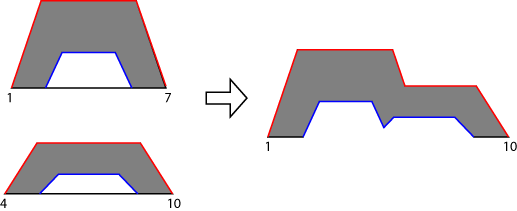For a type-2 Sugeno system, the aggregate fuzzy set is derived using the following steps:

1. Sort the rule output levels (zi) from all the rules into ascending order. These output level values define the universe of discourse for the aggregate type-2 fuzzy set.

2. For each output level, define the UMF value using the maximum firing range value from the corresponding rule.

3. For each output level, define the LMF value using the minimum firing range value from the corresponding rule.

For example, suppose you have a type-2 Sugeno system with seven rules. Further, assume these rules have the following output levels and firing range limits.

RuleOutput Level (z)Minimum Firing ValueMaximum Firing Value
16.30.10.5
24.90.40.5
31.60.30.5
45.80.50.7
55.40.20.6
60.70.50.8
73.20.20.7

The following figure shows the aggregated type-2 fuzzy set with its associated UMF (red) and LMF (blue).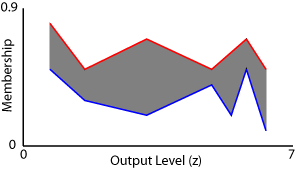#### Type Reduction and Defuzzification

To find the final crisp output value for the inference process, the aggregate type-2 fuzzy set is first reduced to an interval type-1 fuzzy set, which is a range with lower limit cL and upper limit cR. This interval type-1 fuzzy set is commonly referred to as the centroid of the type-2 fuzzy set. In theory, this centroid is the average of the centroids of all the type-1 fuzzy sets embedded in the type-2 fuzzy set. In practice, it is not possible to compute the exact values of cL and cR. Instead, iterative type-reduction methods are used to estimate these values.

For a given aggregate type-2 fuzzy set, the approximate values of cL and cR are the centroids of the following type-1 fuzzy sets (green).Mathematically, these centroids are :

`$\begin{array}{l}{c}_{L}\approx \frac{{\sum }_{i=1}^{L}{x}_{i}{\mu }_{umf}\left({x}_{i}\right)+{\sum }_{i=L+1}^{N}{x}_{i}{\mu }_{lmf}\left({x}_{i}\right)}{{\sum }_{i=1}^{L}{\mu }_{umf}\left({x}_{i}\right)+{\sum }_{i=L+1}^{N}{\mu }_{lmf}\left({x}_{i}\right)}\\ {c}_{R}\approx \frac{{\sum }_{i=1}^{R}{x}_{i}{\mu }_{lmf}\left({x}_{i}\right)+{\sum }_{i=R+1}^{N}{x}_{i}{\mu }_{umf}\left({x}_{i}\right)}{{\sum }_{i=1}^{R}{\mu }_{lmf}\left({x}_{i}\right)+{\sum }_{i=R+1}^{N}{\mu }_{umf}\left({x}_{i}\right)}\end{array}$`

Here:

• N is the number of samples taken across the output variable range, specified using `evalfisOptions`.

• xi is the ith output value sample.

• μumf is the upper membership function.

• μlmf is the lower membership function.

• L and R are switch points that are estimated by the various type-reduction methods. For a list of supported methods, see Type-Reduction Methods.

For both Mamdani and Sugeno systems, the final defuzzified output value (y) is the average of the two centroid values from the type reduction process.

`$y=\frac{{c}_{L}+{c}_{R}}{2}$`

### Type-Reduction Methods

Fuzzy Logic Toolbox software supports four built-in type-reduction methods. These algorithms differ in their initialization methods, assumptions, computational efficiency, and terminating conditions.

To set the type-reduction method for a type-2 fuzzy system, set the `TypeReduction` property of the `mamfistype2` or `sugfistype2` object.

Method`TypeReduction` property ValueDescription
Karnik-Mendel (KM) `"karnikmendel"`

First type-reduction method developed

Enhanced Karnik-Mendel (EKM) `"ekm"`

Modification of the Karnik-Mendel algorithm with an improved initialization, modified termination condition, and improved computational efficiency

Iterative algorithm with stop condition (IASC) `"iasc"`

Iterative improvement to brute force methods

Enhanced iterative algorithm with stop condition (EIASC) `"eiasc"`

Improved version of the IASC algorithm

In general, the computational efficiency of these methods improve as you move down the table.

You can also use your own custom type-reduction method. For more information, see Build Fuzzy Systems Using Custom Functions.

 Mendel, J.M., H. Hagras, W.-W. Tan, W.W. Melek, and H. Ying, Introduction to Type-2 Fuzzy Logic Control. Hoboken, NJ. Wiley and IEEE Press (2014)

 Karnik, N.N. and J.M. Mendel, "Centroid of a type-2 fuzzy set," Information Sciences, vol. 132, pp. 195-220. (2001)

 Wu, D. and J.M. Mendel, "Enhanced Karnik-Mendel algorithms," IEEE Transactions on Fuzzy Systems, vol. 17, pp. 923-934. (2009)

 Duran, K., H. Bernal, and M. Melgarejo, "Improved iterative algorithm for computing the generalized centroid of an interval type-2 fuzzy set," Annual Meeting of the North American Fuzzy Information Processing Society, pp. 190-194. (2008)

 Wu, D. and M. Nie, "Comparison and practical implementations of type-reduction algorithms for type-2 fuzzy sets and systems," Proceedings of FUZZ-IEEE, pp. 2131-2138 (2011)